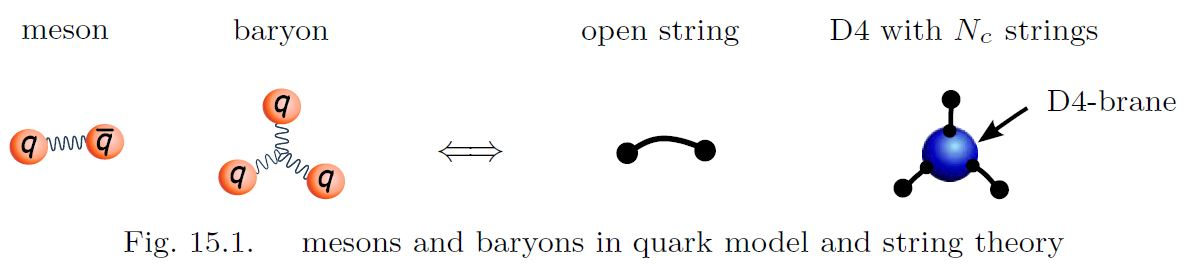Contents

# Contents

## Idea

A brane worldvolume $\phi \colon \Sigma \longrightarrow X$ is said to wrap a cycle $c \in H_{\dim(\Sigma)}(X)$ in spacetime $X$ when the pushforward $\phi_\ast [\Sigma] \in H_\bullet(X)$ of the fundamental class of $\Sigma$ is the class, $[c]$, of the given cycle in $X$. If the pushforward is a multiple of $[c]$, then the brane is said to wrap $c$ multiple times.

## Examples

Via the identification of D-brane charge in K-theory, the K-theoretic McKay correspondence formalizes how D-branes wrap the fundamental cycles in the blow-up resultion of an ADE-singularity (Gonzalez-Sprinberg & Verdier 83)graphics grabbed from Sugimoto 16